# Tangent-squared function

This article is about a particular function from a subset of the real numbers to the real numbers. Information about the function, including its domain, range, and key data relating to graphing, differentiation, and integration, is presented in the article.
View a complete list of particular functions on this wiki
For functions involving angles (trigonometric functions, inverse trigonometric functions, etc.) we follow the convention that all angles are measured in radians. Thus, for instance, the angle of$90\,^\circ$ is measured as$\pi/2$.

## Definition

This function is defined as the composite of the square function and the tangent function. Explicitly, it is the function: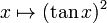$x \mapsto (\tan x)^2$$(\tan x)^2$ is written as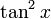$\tan^2x$ for shorthand.

## Key data

Item Value
Default domain all real numbers except the odd integer multiples of$\pi/2$.
range$[0,\infty)$, i.e.,$\{ y \mid y \ge 0 \}$. All nonnegative reals.
no absolute maximum value; absolute minimum value of 0
period$\pi$, i.e.,$180\,^\circ$.
local maximum values and points of attainment No local maximum values
local minimum values and points of attainment 0 at all integer multiples of$\pi$.
points of inflection (both coordinates) None
vertical asymptotes at all odd multiples of$\pi/2$, with the function going to$+\infty$ from both directions in each case.
derivative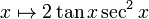$x \mapsto 2\tan x \sec^2x$
antiderivative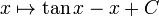$x \mapsto \tan x - x + C$. Note that the$C$ value must be constant within each interval between consecutive odd multiples of$\pi/2$, but can be different in different intervals. The domain overall is not connected.
interval description based on increase/decrease and concave up/down For each integer$n$:
decreasing and concave up from$n\pi - \pi/2$ to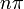$n\pi$
increasing and concave up from$n\pi$ to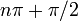$n\pi + \pi/2$.

## Differentiation

### First derivative

The first derivative can be computed by combining the chain rule for differentiation and knowledge of the derivatives of the square function and the tangent function:$\frac{d}{dx}[\tan^2x] = \frac{d}{d(\tan x)}[\tan^2x] \frac{d(\tan x)}{dx} = (2\tan x)(\sec^2 x)$

## Integration

### First antiderivative

We use the identity:$\tan^2x + 1 = \sec^2x$

Using this, we rewrite: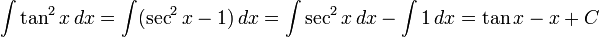$\int \tan^2x \, dx = \int (\sec^2x - 1) \, dx = \int \sec^2 x \, dx - \int 1 \, dx = \tan x - x + C$

where we use that the tangent function is an antiderivative for the secant-squared function

### Second antiderivative

We can antidifferentiate the function one more time:$\int \int \tan^2x \, dx \, dx = \int (\tan x - x + C)\, dx = -\ln|\cos x| - x^2/2 + Cx + C_0$

### Higher antiderivatives

It is not possible to compute higher antiderivatives in terms of elementary functions, but we can compute them using the polylogarithm.

### Integration of products with polynomials

Using integration by parts, we know that if it is possible to integrate a function$k$ times, it is also possible to use that information to integrate$x^{k-1}$ times the function. Thus, from the above, we can integrate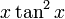$x\tan^2x$ within elementary functions. However, it is not possible to integrate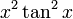$x^2\tan^2x$ within elementary functions.

Explicitly, we have:$\int x\tan^2x \, dx = x \int \tan^2x \, dx - \int \int \tan^2x \, dx \, dx = x(\tan x - x) + \ln|\cos x| + x^2/2 + C = x\tan x + \ln|\cos x| - x^2/2 + C$